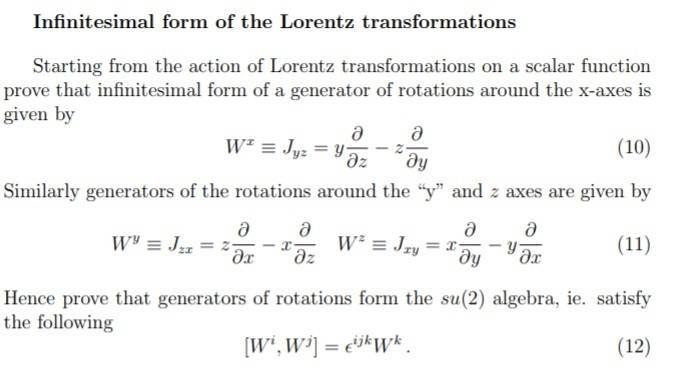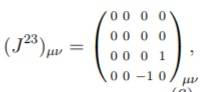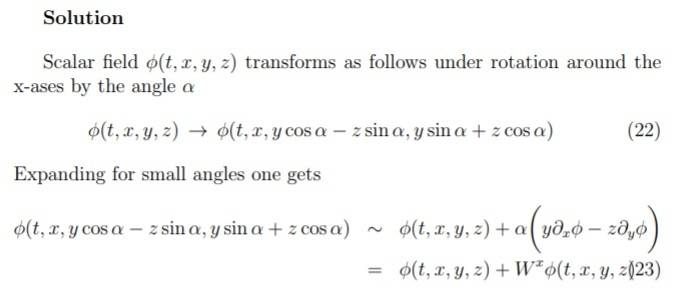# Infinitesimal form of the Lorentz Transformation

## Homework Statement

attached:## Homework Equations

where ##J_{yz} ## is## The Attempt at a Solution

[/B]
In a previous question have exponentiated the generator ##J_{yz}## to show it is the generator of rotation around the ##x## axis via trig expansions

so ##\Phi(t,x,y,z) \to \Phi(t,x,y cos \alpha - z sin \alpha, y sin \alpha + z cos \alpha ) ## and so via small angle expansions have:

##y \to ( y(1-\frac{\alpha^2}{2}+O(\alpha^4))-z(\alpha-\frac{\alpha^3}{3!}+O(\alpha^5))) ##
and
## z\to ( ( z(1-\frac{\alpha^2}{2}+O(\alpha^4))+y(\alpha-\frac{\alpha^3}{3!}+O(\alpha^5)))##

I am unsure now how expand. I thought perhaps a taylor expansion in multivariables - y and z- was the idea, but I can't see how you would arrive at the answer attached with this:Any tips appreciated.ta.

fresh_42
Mentor

## Homework Statement

attached:View attachment 227270

## Homework Equations

where ##J_{yz} ## is View attachment 227273

## The Attempt at a Solution

[/B]
In a previous question have exponentiated the generator ##J_{yz}## to show it is the generator of rotation around the ##x## axis via trig expansions

so ##\Phi(t,x,y,z) \to \Phi(t,x,y cos \alpha - z sin \alpha, y sin \alpha + z cos \alpha ) ## and so via small angle expansions have:

##y \to ( y(1-\frac{\alpha^2}{2}+O(\alpha^4))-z(\alpha-\frac{\alpha^3}{3!}+O(\alpha^5))) ##
and
## z\to ( ( z(1-\frac{\alpha^2}{2}+O(\alpha^4))+y(\alpha-\frac{\alpha^3}{3!}+O(\alpha^5)))##

I am unsure now how expand. I thought perhaps a taylor expansion in multivariables - y and z- was the idea, but I can't see how you would arrive at the answer attached with this:View attachment 227272

Any tips appreciated.ta.
It is far easier, although not really short. Simply apply ##[W^i,W^j]=W^iW^j-W^jW^i## to a function ##f(x,y,z)##.

vela
Staff Emeritus
Homework Helper
There's a typo in the solution you attached. Is that what's confusing you?

strangerep
Any tips appreciated.ta.
I'm not sure whether this is giving too much of a hint on the first round, but here it is...

For a 1-parameter coordinate transformation ##x^\mu \to x'^\mu(x,\alpha)##, where ##\alpha## is the parameter, and ##\alpha=0## corresponds to the identity transformation, the generator ##G## can be computed from the following formula:$$G ~=~ \left. \frac{\partial x'^\mu}{\partial\alpha} \right|_{\alpha=0} \, \frac{\partial}{\partial x^\mu} ~~,$$(with implicit summation over the index ##\mu##).

HTH.

I'm not sure whether this is giving too much of a hint on the first round, but here it is...

For a 1-parameter coordinate transformation ##x^\mu \to x'^\mu(x,\alpha)##, where ##\alpha## is the parameter, and ##\alpha=0## corresponds to the identity transformation, the generator ##G## can be computed from the following formula:$$G ~=~ \left. \frac{\partial x'^\mu}{\partial\alpha} \right|_{\alpha=0} \, \frac{\partial}{\partial x^\mu} ~~,$$(with implicit summation over the index ##\mu##).

HTH.

okay thanks
ive never come across this before, could someone give me a brief summary of some background or point me to a link?

(also may i ask who takes credit for the last quote in your sig :P ? )

strangerep
[...] could someone give me a brief summary of some background [...]
You just have to fill in a couple of steps in the solution you posted (which, as vela said, does indeed contain a typo).

In a little more detail, express ##\Phi'\Big(x^\mu(\alpha)\Big)## as a Taylor series in ##\alpha## (to 1st order is enough). Then use the chain rule to re-express ##d\Phi'/d\alpha## in terms of ##\partial\Phi'/\partial x^\mu## (since ##\Phi'## only depends on ##\alpha## indirectly, via the dependence of ##x'^\mu## on ##\alpha##).

(also may i ask who takes credit for the last quote in your sig :P ? )
That's mine. But "credit" is probably the wrong word.

•binbagsss
fresh_42
Mentor
I don't think that a Taylor series is needed. Just calculate things like ##\frac{\partial }{\partial x_\mu}(x_v f_\nu(x))## etc.

strangerep
I don't think that a Taylor series is needed. Just calculate things like ##\frac{\partial }{\partial x_\mu}(x_v f_\nu(x))## etc.
Huh?fresh_42
Mentor
Huh?I mean, we have differential operators and the multiplication times ##x_\nu##. As we are only interested in the commutator relations of them, we can simply take any twice differentiable function in three variables and calculate what these operators do. No need for any power series, the manifold itself, or a parameter. Simply differentiate: (I set ##\partial_x := \dfrac{\partial}{\partial x}\; , \;etc.)##
$$[W^x,W^y](f)=(y\partial_z - z \partial_y)(z\partial_x - x\partial_z)(f) - (z\partial_x - x\partial_z)(y\partial_z - z \partial_y)(f) = \ldots$$
I would further set ##f_x:=\partial_x (f)\, , \,etc.## to keep the workload low, but there is nothing else needed than good old Leibniz rule. It's trivial.

strangerep
No need for any power series,
Oh, I see. You're answering a different part of the question.

I was explaining how to compute the generator corresponding to a particular (finite) coordinate transformation. You're explaining how to calculate commutator(s) between generators.

Last edited:
fresh_42
Mentor
Oh, I see. You're answering a different part of the question.

I was explaining how to compute the generator(s) corresponding to a particular (finite) coordinate transformation. You're explaining how to calculate commutator(s) between generators.
Uh, o.k., I only concentrated on the image, and then I didn't understand where the entire rest was necessary for. How to get the tangents versus how do they behave or vice versa.